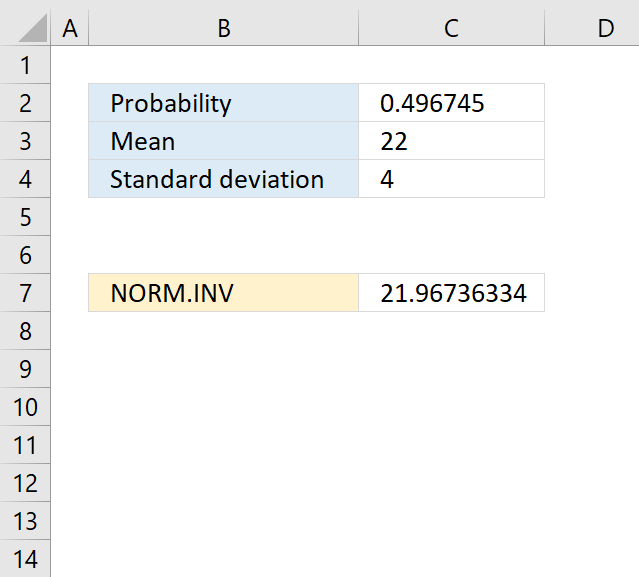Author: Oscar Cronquist Article last updated on April 16, 2018The NORM.INV function calculates the inverse of the normal cumulative distribution for a given mean and standard deviation.

Formula in cell C7:

=NORM.INV(C2,C3,C4)

### Excel Function Syntax

NORM.INV(probabilitymeanstandard_dev)

### Arguments

 probability Required. A probability corresponding to the normal distribution. mean Required. The average of the distribution. standard_dev Required. The standard deviation of the distribution.